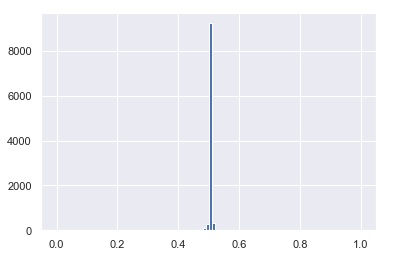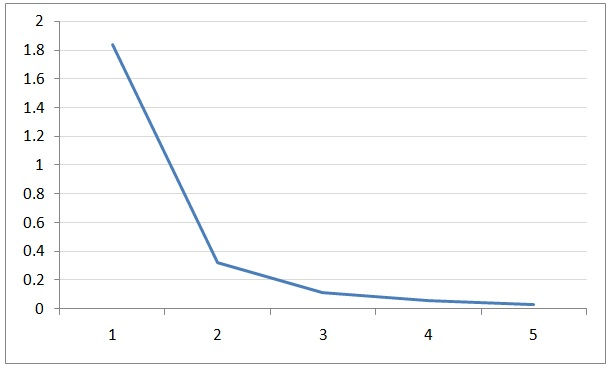top of page
Search
• riccardo

# The illusion of control _ Central limit theorem

Updated: Dec 26, 2018Sometimes, the simpler the tool, the harder it is to master it.

In management, investment and business in general, one of the tool we often rely on – even if not consciously or explicitly – is the central limit theorem. In short, it guarantees that sums and averages of variables (random) converge to the Gaussian distribution, no matter the specific distribution of the variables – as long as they all have the same distribution. The reader can find formal definitions; I prefer to show examples below. Again, please note that even though the reader may be not really computing calculations and numbers, he / she may be still relaying on this concept very often.

I try below to reach an intuitive understanding of some of the major pitfalls of that common approach to problem solving; however, I am far from completely and immediately handling it, that is why I often rely on simulation. I experienced those pitfalls while practicing with some machine learning programming and options trading but, I then perceived the same dynamics in many businesses parameters – mainly, numbers on sales and costs. Possible red flags in business are cases where one or two customers or products represent the majority of the sales – related to the Pareto distribution.

Simple example of the central limit theorem; a fair coin: the distribution of such coin would be something called the Bernoulli distribution. The distribution is discrete with only two possible values: probability 0.5 of ending up head (assigned value 0) and probability 0.5 of ending up tail (assigned value 1). That distribution is clearly different from a Gaussian one and it is something similar to the picture below considering p and 1-p both equal to 0.5 in this case:https://www.probabilitycourse.com/chapter3/3_1_5_special_discrete_distr.php

By flipping the coin 10,000 times and recording the changing average of the outcome – sum of 0s and 1s divided by the number of flips at each draw – we would get something like the following:The average would rapidly converge to the expected value 0.5. More importantly, as the central limit theorem predicts, if we extract groups of n random draws from the total 10k and we plot the histogram of the average of each one of those groups of n draws – let’s choose n=10 – we would get something like this:Here we can see that the average of each group of 10 draws behaves like a Gaussian random variable. Basically, the majority of the groups of 10 draws have an average value close to the expected 0.5 and a gaussian deviation from it. That is a very important result because it means that the theorem allows us to exploit useful Gaussian tools even with random variables not normally distributed; Bernoulli distributed in this case – the reader can also try to draw random variables from a Uniform or different distribution.

However, the central limit theorem is based on specific assumptions; in particular one of them considers the identically distributed random variables all having finite variance – therefore standard deviation. We can consider a fair coin with head corresponding to the value 0 and tail corresponding to 1 having mean = 0.5 and variance = 0.25 – therefore, standard deviation of sqrt(0.25) = 0.5 and finite. That is in part why the theorem works in this case.

However, as per other posts, many real-life situations are characterized by probability distributions not having finite variance. Examples could be the Pareto or Power Law distribution; even though the exact behavior would depend on their parameters – I focus again on the Power Law distribution just to give a possible continuum in case the reader is following other posts on this channel but, the discussion should be generalized to entire classes of similar distributions not having finite variance. What may appear to be a small difference among parameters may cause major differences in behavior. It is worth to point out that distributions like the Pareto and Power Law may describe phenomena like the stock-market returns, the distribution of wealth, the R&D costs of a technology company and so on… therefore, they may characterize many real-life scenarios.

Below I show three Pareto distributions corresponding to slightly different parameters, yet completely different behaviors – please, allow me not to re-scale each distribution:Parameter alpha = 2.5Parameter alpha = 1.5Parameter alpha = 0.5

The danger comes from the fact that sometimes data appear to be normally (Gaussian) distributed while, a deeper study may reveal that the tails of the distributions act like one of the distributions above, implying very important consequences. Below, a qualitative example of how an apparent Gaussian distribution may hide “strange” tail dynamics; in those cases we may think of applying common “Gaussian” methodologies, potentially obtaining very wrong results:The three distributions shown above have completely different behaviors characterized by different means and standard deviations; in particular, while the first is pretty normal the second has undefined variance and the third has undefined variance and mean. By repeating the same simulation of the coin-flips but, drawing from the three distributions above, we would get the results shown below. Again, the first picture represents the changing average as we draw from the distribution till we reach 10k trials while, the second picture represents the distribution of the averages of groups of 10 random samples among the 10k:Distribution 1, alpha 2.5: picture below -> mean=0.66 (stable -> close to the picture above), stdDev=0.4 (stable)Distribution 2, alpha 1.5: picture below -> mean=2 (stable -> close to the picture above), stdDev = 8 (starts going off)Distribution 3, alpha 0.5: Picture below -> mean=undefined, stdDev=undefined

As the reader can see form the numbers in the captions of each picture, in the first case we have a stable mean and variance, in the second case we only have a stable mean and in the third case we completely lose control of numbers. Those very different dynamics may be hard to predict just by looking at the three initial distributions we drew numbers from.

Note for the interested reader: numbers in the third case reach high values and an example is shown below; however, the thing to note is that those numbers are highly unstable and change from iteration to iteration while in the first two cases (alpha equal to 2.5 and 1.5) they stay around fix values.Distribution 3, alpha = 0.5: example showing the full range of numbers. By repeating the simulation numbers would change because of the "instability" given by the particular distribution admitting possible very high numbers.

Now, assume that a particular business had some parameters apparently normally distributed but, with real tail-characteristics similar to the third case above; we would have no control of numbers while we may be still relying on computing averages and deviations for our forecasts. Also, please note that in real life scenarios data are not completely available and having a thorough understanding of the real distribution may be very difficult. Therefore, intuitive reasoning could be precious; for example in physics and engineering, knowing that a particular parameter is subject to some conservation-laws may help us define the ranges and distribution but, in other business-applications that may be not the case.

This simple and basic experiment shows how important is to be suspicious about the data we are handling. It is important to understand that even if we determined that our numbers acted as per the third case above, we would still have many options to implement; however, it would all start with an open mind versus scenarios very different from the ones we are used to.

Main image's tag:

bottom of page